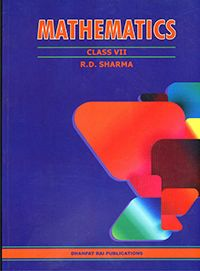# Mathematics Class VII by R. D. Sharma

265.00 252.00

1. Integers

2. Fractions

3. Decimals

4. Rational numbers

5. Operations on Rational numbers

6. Exponents

7. Algebraic Expressions

8. Linear Equations in one variable

9. Ratio and proportion

10. unitary method

11. Percentage

12. Profit and loss

13. Simple interest

14. Lines and angles

15. Properties of triangles

16. Congruence

17. Constructions

18. Symmetry

19. Visualising solid shapes

20. Mensuration – I

21. Mensuration – II

22. Data Handling I (Collection and Organisation of Data)

23. Data Handling II (Central Values)

24. Data Handling III (Construction of bar graphss)

25. Data Handling IV (Probability)